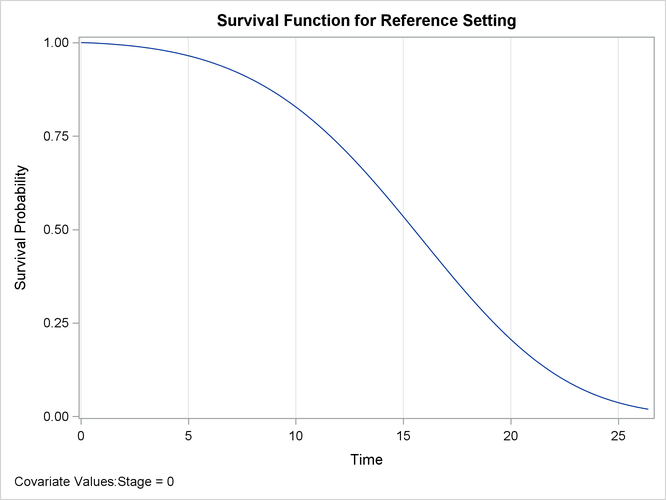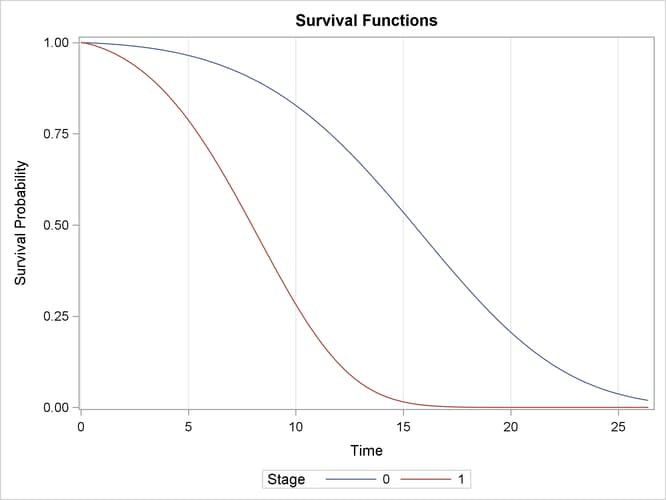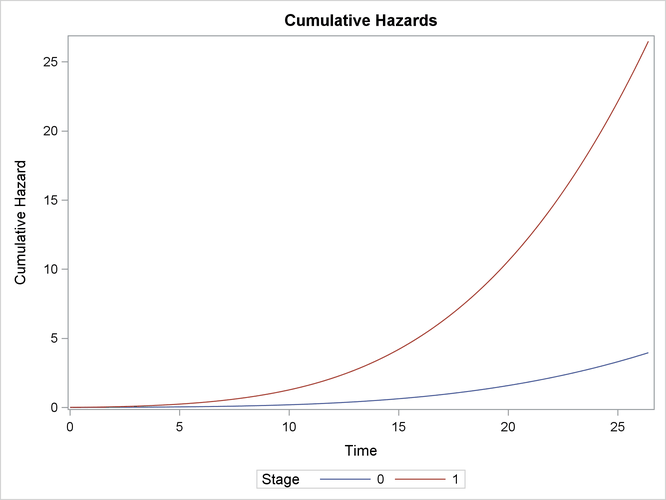# The ICPHREG Procedure

### Example 63.2 Plotting Predicted Survival and Cumulative Hazard Functions

This example illustrates how to plot the predicted survival and cumulative hazard functions for specified covariate patterns.

The following statements request a plot of the estimated baseline survival function:

```ods graphics on;
proc icphreg data=hiv plot=surv;
class Stage / desc;
model (Left, Right) = Stage / basehaz=splines;
run;
```

Output 63.2.1 shows the predicted survival curve at the reference level.

Output 63.2.1: Estimated Survival Curve for the Reference SetTo produce curves for general covariate patterns, you can specify the COVARIATES= option in the BASELINE statement. The following statements create observations for two levels of `Stage` and plot the corresponding predicted curves:

```data cov;
Stage=0; output;
Stage=1; output;
run;

proc icphreg data=hiv plot=surv;
class Stage / desc;
model (Left, Right) = Stage / basehaz=splines;
baseline covariates=cov;
run;
```

Under the proportional hazards assumption, the two curves do not cross each other. As shown in Output 63.2.2, patients at Stage 1 have much lower survival rates than patients at Stage 0.

Output 63.2.2: Predicted Survival Curves for Specified Covariate PatternsThe following statements request a plot of the predicted cumulative hazard functions for the two levels of `Stage`:

```proc icphreg data=hiv plot=cumhaz;
class Stage / desc;
model (Left, Right) = Stage / basehaz=splines;
baseline covariates=cov;
run;
```

Output 63.2.3 shows the plot.

Output 63.2.3: Predicted Cumulative Hazards for Specified Covariate Patterns